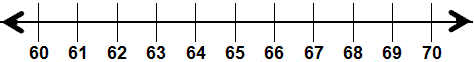Skill 16B Estimate to see if Answer is Reasonable 1. First subtract. 2. Next, estimate each number below by rounding each number to the nearest ten. Write each estimated number in the box by each arrow. 3. You may use the number line to help you round to the nearest ten. 4. Is your estimated difference close to the exact difference?